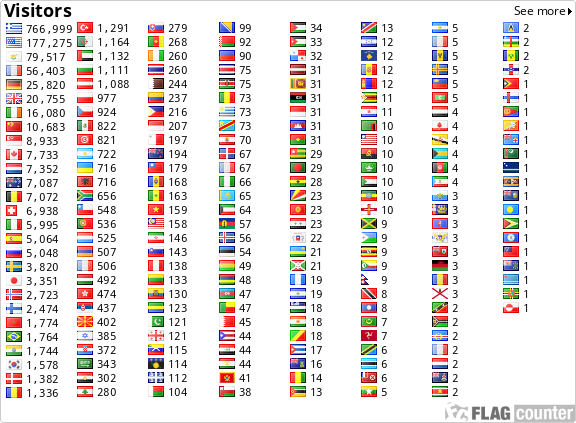﻿Simplicity and Reality - N. Lygeros

Simplicity and Reality

N. Lygeros

Translated from the Greek by Athena Kehagias

 The general tendency of the modeling of a phenomenon is to find a model with a simple structure that can reproduce the initial complexity. This principle constitutes the basis of science, as defined by Leibniz, as the formalism of the mental scheme became necessary. From this principle, the idea of the simplicity of the phenomenon does not necessary derive. Historical results in the field of physics, especially as the magnetic moment of the electron and the Feynman diagrams, are proving that, the initial models are simplistic. But simplicity does not mean simplemindedness:. The understanding of a phenomenon as observed aptly by Chaitin it is also information compression .The power of the model structure is able to absorb the frame of the complexity of the model. Moreover, the field of fractional analysis demonstrated that even relatively simple principles can produce great complexity.The examples of the Poincare section or of the strange attractor of Milnor are quite sufficient.Therefore, there is no need to raise the issue of simplifying in tis common sense, because it has no sense as a science. Recent discoveries in the gnostic field, contribute to the assumption that there are correlations between brain structure and evolution of genetic algorithms, neural networks, and complex networks in general.This revises the idea of creativity of a simple model. And we observe that, there is a need to invent simple models, in regards to their structure, which contain a large complexity in terms of their evolutionary behaviour. This new approach deepens the ideology of Leibniz, but also that of Einstein's, yet the overall problem of negation remains, as it is based on Godel's proof of the total codification of a system comprehensive .Because the modelisation is a form of encoding, which could only in very specific cases be total.So, this axiomatic principle of freedom of choice, creates a context where intelligence is necessary when there is no perfect information existent.The same reason explains the development of lateral thinking strategies,where there are structures which could not be understood algorithmically. These mental schemes, through Turing's discoveries, open new horizons in the field of theoretical modelisation.With the problem of of Eco's open structures and the deep structures of Chomsky, the complexity of simplicity becomes an effective tool for the investigation of the nature of reality, which could be interpreted as a simple structure that creatively self explains itself.But by the same token, through the generalized theorem of Chaitin-Gödel-Turing, we know that, there are non processable model phenomena which should be included as axioms in the total elegant theories.Opus